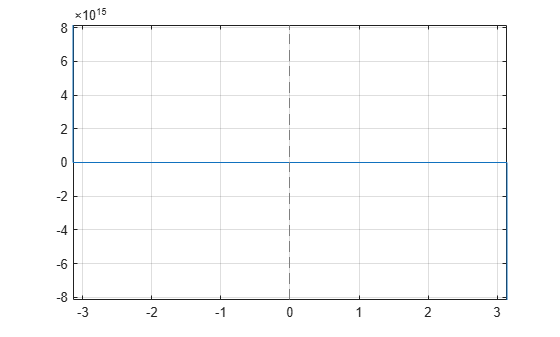# cot

Symbolic cotangent function

## Syntax

``cot(X)``

## Description

example

``cot(X)` returns the cotangent function of `X`.`

## Examples

### Cotangent Function for Numeric and Symbolic Arguments

Depending on its arguments, `cot` returns floating-point or exact symbolic results.

Compute the cotangent function for these numbers. Because these numbers are not symbolic objects, `cot` returns floating-point results.

`A = cot([-2, -pi/2, pi/6, 5*pi/7, 11])`
```A = 0.4577 -0.0000 1.7321 -0.7975 -0.0044```

Compute the cotangent function for the numbers converted to symbolic objects. For many symbolic (exact) numbers, `cot` returns unresolved symbolic calls.

`symA = cot(sym([-2, -pi/2, pi/6, 5*pi/7, 11]))`
```symA = [ -cot(2), 0, 3^(1/2), -cot((2*pi)/7), cot(11)]```

Use `vpa` to approximate symbolic results with floating-point numbers:

`vpa(symA)`
```ans = [ 0.45765755436028576375027741043205,... 0,... 1.7320508075688772935274463415059,... -0.79747338888240396141568825421443,... -0.0044257413313241136855482762848043]```

### Plot Cotangent Function

Plot the cotangent function on the interval from $-\pi$ to $\pi$.

```syms x fplot(cot(x),[-pi pi]) grid on```### Handle Expressions Containing Cotangent Function

Many functions, such as `diff`, `int`, `taylor`, and `rewrite`, can handle expressions containing `cot`.

Find the first and second derivatives of the cotangent function:

```syms x diff(cot(x), x) diff(cot(x), x, x)```
```ans = - cot(x)^2 - 1 ans = 2*cot(x)*(cot(x)^2 + 1)```

Find the indefinite integral of the cotangent function:

`int(cot(x), x)`
```ans = log(sin(x))```

Find the Taylor series expansion of `cot(x)` around ```x = pi/2```:

`taylor(cot(x), x, pi/2)`
```ans = pi/2 - x - (x - pi/2)^3/3 - (2*(x - pi/2)^5)/15```

Rewrite the cotangent function in terms of the sine and cosine functions:

`rewrite(cot(x), 'sincos')`
```ans = cos(x)/sin(x)```

Rewrite the cotangent function in terms of the exponential function:

`rewrite(cot(x), 'exp')`
```ans = (exp(x*2i)*1i + 1i)/(exp(x*2i) - 1)```

### Evaluate Units with `cot` Function

`cot` numerically evaluates these units automatically: `radian`, `degree`, `arcmin`, `arcsec`, and `revolution`.

Show this behavior by finding the cotangent of `x` degrees and `2` radians.

```u = symunit; syms x f = [x*u.degree 2*u.radian]; cotf = cot(f)```
```cotf = [ cot((pi*x)/180), cot(2)]```

You can calculate `cotf` by substituting for `x` using `subs` and then using `double` or `vpa`.

## Input Arguments

collapse all

Input, specified as a symbolic number, variable, expression, or function, or as a vector or matrix of symbolic numbers, variables, expressions, or functions.

collapse all

### Cotangent Function

The cotangent of an angle, α, defined with reference to a right angled triangle is

.The cotangent of a complex argument α is

`$\text{cot}\left(\alpha \right)=\frac{i\left({e}^{i\alpha }+{e}^{-i\alpha }\right)}{\left({e}^{i\alpha }-{e}^{-i\alpha }\right)}\text{\hspace{0.17em}}.$`

.